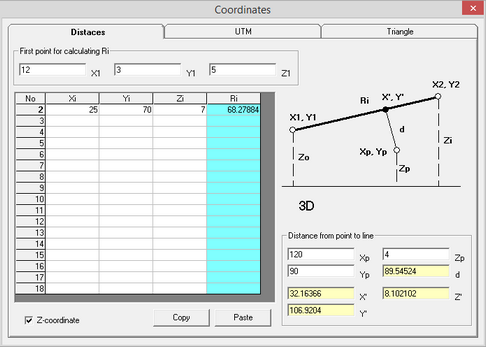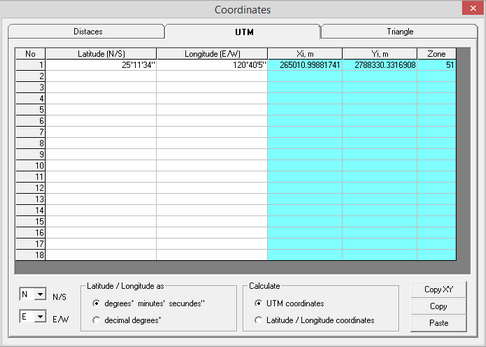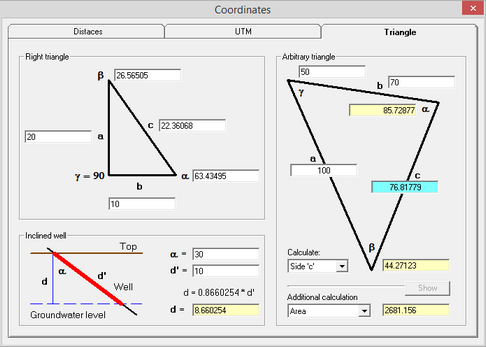﻿ Help > Coordinates

# Coordinates

The dialog window "Coordinates" assists in calculation of distances between locations with known coordinates and for coordinates conversion between UTM system and latitude/longitude degrees. Calculations are done through a series of tables with copy and paste options.

The dialog window is opened from menu "Help > Coordinates...".Tab examples of the dialog window "Coordinates"

Explanatory table

 Tab "Distances" Calculates distances between two points in space Frame «First point for calculating Ri» Coordinates of the first point (fields «X1», «Y1» и «Z1») for distance calculations. For distance calculations between a point and a straight line (frame «Distance from point to line») this is the first point (X1, Y1) of the straight line on the sketch of the dialog window Check box "Z-coordinate" Includes vertical dimension (coordinate Z) in calculations. When this box is not checked, distances are calculated on a plane surface (topography is neglected). With the checked box calculations between two points are done in space (3-dimensions) Table Coordinates of the 2-nd point are entered in this table. Each row of the table can have coordinates of a different point. Moving the cursor on a specific row of the table results in automatic calculation of the distance for this row in the highlighted cell. Frame «Distance from point to line» Calculates the distance (field «d») from the first point (fields «Xp», «Yp» and «Zp») to the straight line as presented on the sketch of the dialog window. The straight line is determined by the two points: •First point for calculating Ri and •the point with coordinates in the table. The straight line direction is changing when the cursor moves through the table. The fields «X'», «Y'» и «Z'» show the calculated coordinates of the intersection point between the straight line and its perpendicular passing by the point  «Xp», «Yp» и «Zp». When one of the coordinates «X'», «Y'» or «Z'» is entered, the other two coordinates are automatically calculated. In the latter case, coordinates (X', Y', Z') become equal to (Xp, Yp, Zp) and the distance to the straight line (d) becomes 0. Tab "UTM" Converts coordinates from meters in UTM system to degrees and reverse. The algorithm for the conversion is sourced from the website: http://www.movable-type.co.uk/scripts/latlong-utm-mgrs.html Table Coordinates either in degrees or in meters are entered in this table. There are limitations on values that can be entered. If input values fall outside a pre-determined range, the program will automatically correct them. Context info about the pre-determined range of values is displayed, when the cursor is placed on a specific column. List "N/S" Selection between Northern and Southern hemispheres. Changing selection results in recalculation of table values. List "E/W" Selection between Eastern and Western hemispheres. Changing selection results in recalculation of table values. Frame "Latitude / Longitude" Selection of format for coordinates in degrees (Latitude/Longitude coordinates). Coordinates can be entered either in the format of degrees-minutes-seconds (for example, 25°10'50" or 0°32'17.92") or in the format of decimal degrees (for example, 25.1805566° or 0.53831° – symbol of degree is not necessary). Changing the selected format results in re-calculation of values in the table. When data are input in the format of degrees-minutes-seconds, the values for degrees, minutes and seconds need to be separated by any symbol (for example, space – 25 10 50). The programm automatically re-formats the entered values. Number of minutes or seconds must be less than 60. Degrees and minutes must be entered as integer numbers, while seconds may be input with decimals. Frame "Calculate" Calculates UTM coordinates (columns Xi, Yi and Zone) from the Latitude/Longitude and reverse. When entering UTM coordinates, it is required to enter the UTM zone (between 1 and 60) Button "Copy XY" Copies columns Xi and Yi on the Clipboard Tab "Тriangle" Determination of sides and angles of triangles Frame «Right triangle» Sides and angles calculations for a right triangle : угол γ = 90°, α + β = 90° Frame «Arbitrary triangle» Sides and angles calculations for any triangle: α + β + γ = 180° List «Calculate» Selects side or angle of the triangle for calculations List «Additional calculation» and text box Calculates triangle area, perimeter, height, median and angle bisector as well as radius of internal and external circles. Button «Show» This button is enabled when "Height", "Median"  "Bisector", "Radius R" or "Radius r" are selected from the List "Additional calculation". After pressing this button, an explanatory sketch appears in the Frame "Arbitrary triangle". Frame «Inclined well» Calculates the vertical distance to the water table in an inclined well using well dip and depth to water in a inclined well.